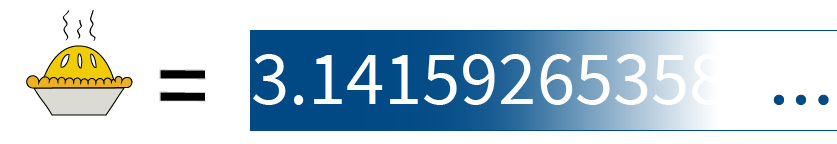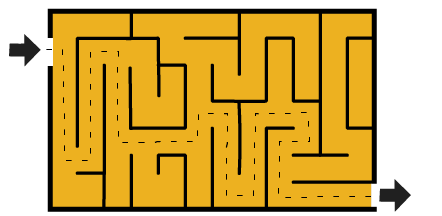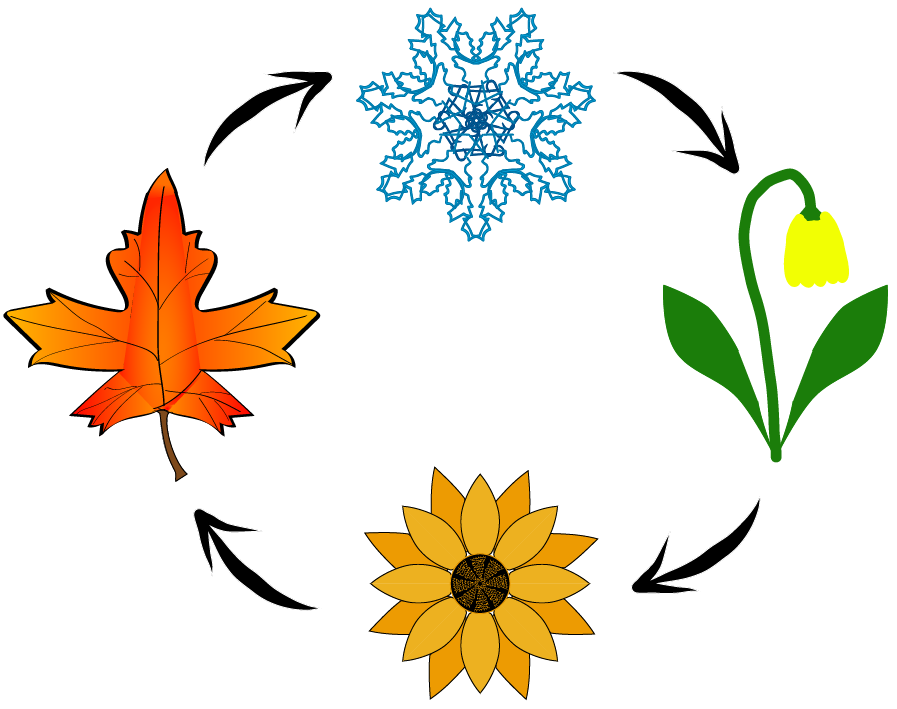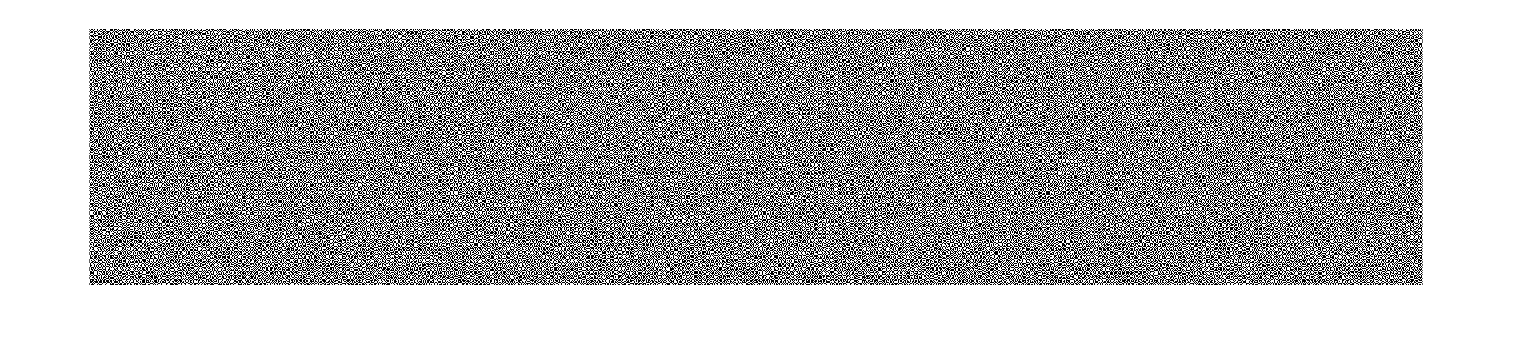## Fundamentals-of-Programming

version 1.0.3 (1.88 MB) by
An overview of programming concepts including arrays, strings, loops, and algorithms with a project.

Updated 12 May 2022

From GitHub

# Fundamentals of ProgrammingCurriculum Module
Created with R2021b. Compatible with R2021b and later releases.

## Description

This curriculum module contains interactive live scripts that teach fundamental concepts and basic terminology related to programming computers. This includes discussion of inputs and outputs, numbers, strings, arrays, branches and loops. This content is broken into six instructional live scripts and one project where live script instructions are paired with a plain code practice script. Solutions are available to instuctors upon request.

These lessons can be used as part of a lecture, as activities in an instructional setting, or as interactive assignments to be completed outside of class.

Get started with the Fundamentals of Programming interactive examples by downloading and unzipping the repository. Then, double-click the FundamentalsofProgramming.prj file inside MATLAB®. From there, you can follow the landing page instructions to get started with the examples. The instructions inside the live scripts will guide you through the exercises and activities. Get started with each live script by running it one section at a time. To stop running the script or a section midway (for example, if a loop is running longer than intended), click the Stop button in the RUN section of the Live Editor tab in the MATLAB Toolstrip.

## Prerequisite Domain Knowledge

This module assumes familiarity with basic mathematics at a high school level. One exercise in programmingData.mlx requires the use of the quadratic formula, for instance. Understanding the chaos game requires locating points on a Cartesian coordinate system and understanding of either vectors or midpoints. Trigonometric functions are used as example functions. With respect to programming knowledge, this module is intended for absolute beginners who have used simple calculators in the past.

## Details

NavigationOverview.mlx This script brings together links and short descriptions of the content in the recommended order of use.

programmingIntroduction.mlx This overview of the concepts of programming also includes discussion of errors, documentation, and other commonly encountered challenges for beginners.Learning Goals:

• Recognize and use the terms input and output
• Recognize, interpret, and respond to messages about syntax errors
• Recognize and interpret comments in code
• Recognize and explain the importance of attention to detail when coding

programmingData.mlx This script introduces `double` and `string` data types through interactive examples and exercises.Learning Goals:

• Recognize and explain that floating point arithmetic does not operate under the same rules as real number arithmetic
• Apply the limitations of floating point arithmetic to computation
• Recognize and use strings to store and communicate textual data
• Recognize and use whitespace in string operations
• Recognize and interpret special characters that are neither text nor numbers
• Recognize and explain simple consequences of the interactions between data types

programmingArrays.mlx This script introduces 1, 2, and 3-D arrays, as well as a brief overview of matrix operations. Applications include both text and image data.

Learning Goals:

• Identify, create, and use vectors containing numbers or text
• Apply vector indexing to extract information from a vector
• Identify, create, and use matrices containing numbers or text
• Apply matrix indexing to extract information from a matrix
• Identify standard file types and the corresponding extensions
• Explain why file type matters and import data from external files
• Recognize and use three dimensional arrays

programmingBranches.mlx This script introduces the concept of conditional branching in the form of if/else branches and switch statements.Learning Goals:

• Recognize and apply if/then branching logic to solve problems
• Recognize and apply switch branching logic to solve problems

programmingLoops.mlx This script introduces the concept of conditional looping in the form of for loops and while loops.Learning Goals:

• Use for loop logic and syntax to write and execute iterative code
• Use while loop logic and syntax to write and execute repetitive code, and decide when it is the correct method

programmingChaosGameProject.mlx

Learning Goals:

• Apply conditional branching and looping logic in context to solve problems
• Explain that different programming solutions to the same problem can run in very different amounts of time
• Identify common file formats and their extensions
• Communicating results by exporting data from a program in a standard, shareable file format

programmingAlgorithms.mlxLearning Goals:

• Explain the concept of an algorithm
• Implement an algorithm presented in pseudocode
• Explain the importance of testing both code and algorithms

chaosGame.m This plain code file presents the basic scaffolding used to build an implementation of the chaos game on a square in `programmingChaosGameProject.mlx`.

CollectedProTips.mlx This live script serves as a collection of notes on using the Live Editor and the MATLAB language in the context of these scripts. All of this material is presented in the other scripts, but this one is used for reference.

Data Files

• `turkeys1.jpg` is used in `programmingIntroduction.mlx` and `programmingArrays.mlx`
• `fileformats.mat` is used in `programmingChaosGameProject.mlx`
• `EncryptedImage.mat` is used in `programmingAlgorithms.mlx`
• `BW_Images.mat` is used in `programmingAlgorithms.mlx`

## Products

MATLAB®

The license for this module is available in the LICENSE.TXT file in this GitHub repository.

## Support

The solutions are available upon instructor request. If you would like to request solutions or have a question, contact the MathWorks online teaching team.

### Cite As

Emma Smith Zbarsky (2022). Fundamentals-of-Programming (https://github.com/MathWorks-Teaching-Resources/Fundamentals-of-Programming/releases/tag/v1.0.3), GitHub. Retrieved .

##### MATLAB Release Compatibility
Created with R2021b
Compatible with R2021a and later releases
##### Platform Compatibility
Windows macOS Linux